## Thursday, 26 December 2013

### Christmas songs

I joined a few musical events before the end of 2013 which include the nutcracker performance (for those who follow don't shoot the pianist, you will know that Nov and Dec are always the time for such performance lol) but I also spent some time on reviewing old and new Christmas songs. By chance I found the game BGM Christmas in the 13th month which is a wonderful piece after all. Now I'm enjoying a succeeder of the song, dancing Christmas in the 13th month's remix from sync. art (I guess it's well known for their touhou remixes) so I hope that you will enjoy them too. Well the above one isn't from sync. art but the best performance among all clips in my opinion that the smooth sound from the viola greatly enhance the dancing that mixes well with the lively going background.

Merry Christmas.

## Thursday, 19 December 2013

### A rare diary

It's long since my last diary written here but this is an important one. As some sort of researcher in the field of computational complexity, I ought to dig the SIMC problem out and make an in depth analysis on it, in particular the complexity classification, and algorithm solving them.

Allow me to brief the problem here.

In the graph, assume every edge has weight 1 that the distance can be calculated accordingly. We also define the distance between edges and vertices. For $e = (u,v) \in E$, $d(e,w) = 0.5 + min(d(u,w), d(v,w))$ and the distance between edges immediately follows. It is clear that the distance measurement is metric. (why?)

For a graph $G = (V,E)$ with two disjoint sets of vertices $V_s, V_c$, define score to be the number of edges closer to $V_c$. i.e. $d(e, V_c) \leq d(e, V_s)$. If the distances are equal it counts as 0.5.

Let's have a function to describe the score: $f(G, V_s, V_c) =~score$.

Instance: $G = (V,E)$ and two disjoint sets of vertices $V_s, V_c \subseteq V$, with some further constraint specified upon the challenge.
Output: Another set $V_{c'}\subseteq V$ that is mutually disjoint with $V_s, V_c$.
Goal: Maximize $f(G,V_s, V_c \cup V_{c'})$.

Maybe you have already heard it before, that Greedy algorithm works really well for this problem. Is it the best solution? It is clear that the greedy algorithm is a polynomial time algorithm. Then is this problem in P? Is it NP? This will be an exciting review of the old problem.

## Sunday, 15 December 2013

### GCD and exponents

LCM (least common multiple) and GCD (greatest common divisor) (for integers as a integral domain) is a relatively easy field that indicates similar behavior for some numbers. In the previous entry I have constructed some related identities using prime factorization, and here I would like to introduce a few related problems that we use the properties of GCD and LCM to solve it.

Definition. For natural numbers $a_1, ..., a_n$, let $[a_1,...,a_n]$ be their LCM and $(a_1,...,a_n)$ be their GCD.

Theorem. For $m,n\in \mathbb{N}$, $mn = [m,n](m,n)$.

Q1. (Korea MO 2013, Day2 Q1) Find all $f: \mathbb{N}\rightarrow \mathbb{N}$ so that $f(mn) = [m,n](f(m), f(n))$.

When you see the above theorem a very natural solution is that $f(m) = m$ but we are asked to find all of them. Is this possible?

Substitute $(m,n) = (1,k)$, we have $f(k) = k(f(1),f(k)) = [1,k](f(1),k(f(1),f(k)))$. Let $(f(1),f(k)) = m$ then $f(k) = k(f(1),km) = km$. It is clear that $m \mid f(1)$ so that $f(k) = k(f(1),km) = kmp$ where $p\in \mathbb{N}$. Clearly, $p=1$ so that $(f(1), k) = 1$. Since $k\in \mathbb{N}$ is arbitrary, we have $f(1) = 1$, and $f(k) = k(f(1),km) = k$.

Here we should notice a fact:

Fact 1. $[a_1,...,a_n] \geq max(a_1,...,a_n)$ and $(a_1,..,a_n) \leq min (a_1,...,a_n)$

GCD is something that decrease when you repetitively take it, and if you can take it arbitrarily that gives the same result, the number is probably 1.

In this question, we put the expansion of $f(k)$ to get a looped GCD brackets, but it can be taken alternatively:

Fact 2. For $m,n\in \mathbb{N}$, $(m,n) = (m,(m,n))$.

The next question is about GCD identity on more variables.

Q2. Find all $(a,b,c) \in \mathbb{N} ^3$ so that $[a,b,c](a,b,c) = abc$

We use the proven identities from the last entry:

Theorem. For $a,b,c\in \mathbb{N}$, $[a,b,c]^2 \prod _{cyc} (a,b) = (a,b,c)^2 \prod _{cyc} [a,b]$.

Now $[a,b,c]^2(a,b,c)^2 = (a,b,c)^4 \prod _{cyc} [a,b]/(a,b) = (a,b,c)^4 \prod _{cyc} (ab/(a,b)^2) = (abc)^2 (a,b,c)^4/ \prod _{cyc} (a,b)^2$ and we have

$[a,b,c](a,b,c) = abc (a,b,c)^2/ \prod_{cyc} (a,b)$

To make the last term 1 it is clear that all numbers must be relatively prime. To see why simply note that $(a,b)^2 \geq (a,b) \geq (a,b,c)$ and equality holds iff $(a,b) = 1$ so that we need $(a,b) = (b,c) = (c,a) = 1$, then $(a,b,c) = 1$.

Clearly all relatively prime triplets satisfies the question.

Sometimes the GCD and LCM notation takes all prime factors into account, but what if we want to consider a particular prime? We can prove the case for a certain prime and by enumerate through the primes we get the desired result as every natural numbers (which is finite) can be factorized into (finite) primes.

Definition. (Highest exponent) For $n\in \mathbb{N}$ and prime $p\in \mathbb{P}$ denote $v_p(n) = k$ if $p^k \mid n$ but $p^{k+1}\nmid n$. If $p\nmid n$ then $v_p(n) = 0$. (In some texts we don't allow 0 but here we just let it be)

Then we can prove GCD results in terms of prime exponents, and the proof is naturally completed by the FTOArithmetic.

Example 1. $v_p([a,b]) = max(v_p(a), v_p(b))$ and $v_p((a,b)) = min (v_p(a), v_p(b))$.

Example 2. Since $max(a,b) + min(a,b) = a+b$, we have $v_p([a,b](a,b)) = v_p([a,b])+v_p((a,b)) = a+b$, which proves the identity $(a,b)[a,b] = ab$.

Example 3. When there are more variables and the equation of interest is cyclic we can WLOG assume the order, here let $v_p(a) =x$, $v_p(b) = y$ and $v_p(c) = z$ and WLOG let $x\geq y\geq z$. Then

$v_p([a,b,c]^2\prod (a,b)) = v_p((a,b,c)^2 \prod [a,b]) = 2x+y+2z$

Here we have an alternate prove for Q2, from mathlinks, using the exponent notation:

Let $v_p(a) =x$, $v_p(b) = y$ and $v_p(c) = z$ and WLOG let $x\geq y\geq z$. The question is equivalent to $x+z = x+y+z$ so that $y = 0 \geq z = 0$, so that $(a,b) = (b,c) = (c,a) = 1$ due to cyclic assumption. Then $(a,b,c) = 1$ and $[a,b,c] = abc$.

Q3. Find all triplets $(a,b,c) \in \mathbb{N}^3$ so that $a+b = (a,b)^2$ in cyclic manner.

We always get trouble when addition steps into questions on GCD. To do this notice that for prime $p\geq 3$, it is impossible that $v_p(a+b) \geq v_p ((a,b))+1$ for all $(a,b) \leftarrow (a,b), (b,c),(c,a)$ (why?) Then we have $(a,b)$ equals to 1 or 4, which allow us to enumerate all cases.

Using the exponent notation we can have a very powerful theorem called the lifting the exponent lemma, that allows us to use the exponent notation to find the highest power of some complicated forms. These can be easily searched so I will not cover it here. It is actually frequently used in current Olympic tournaments!

Three little problems:

1) (Russian MO 1999) Find all bounded sequence $\left\{ a_n \right\} \subseteq \mathbb{N}$ where for all $n\geq 3$, $n\in \mathbb{N}$, $a_n = (a_{n-1}+a_{n-2})/(a_{n-1},a_{n-2})$.

2) (A classical lemma) For $a,m,n\in \mathbb{N}$ and $a\geq 2$ show that $(a^m -1, a^n - 1) = a^{(m,n)}-1$.

3) Describe all the triples $(a,b,c)\in \mathbb{N}^3$ so that $[a,b,c](a,b,c) = \sqrt{abc}$.

## Sunday, 24 November 2013

### A little analysis problem

Recently I've been reading Springer GTM on hyperreals, but I'm not good enough to take any of these into the discussions here. I'm going to discuss a nice little problem here but due to the competitive nature of this question I am not going to disclose the source of it (maybe till the competition being due).

Question. Suppose $\left\{ a_n\right\} \subseteq \mathbb{N}$ strictly increasing. Show that $\sum_{i=1}^{\infty} \frac{a_{i+1}-a_i}{a_i}$ diverges.

A geometric interpretation gives you the conclusion immediately:

Solution. $\sum_{i=1}^{\infty} \frac{a_{i+1}-a_i}{a_i} \geq \sum_{i=1}^{\infty} \sum_{j=a_i}^{a_{i+1}-1} j^{-1} = \sum_{i = a_1}^{\infty} i^{-1}$

That clearly converges as indicated by the comparison test since the last one is a partial harmonic series. Please note that the above expression should be avoided as the $\geq$ sign is vague due to the divergence nature of the series.

Now...

Question 2. Suppose $\left\{ a_n\right\} \subseteq \mathbb{R}^+$ strictly increasing. Will $\sum_{i=1}^{\infty} \frac{a_{i+1}-a_i}{a_i}$ converge?

Extending the above proof to $\mathbb{R}$ seems to be a nice idea. To do this we shall compare the term $\frac{a_{i+1}-a_i}{a_i}$ with the integral $\int ^{a_{i+1}}_{a_i} x^{-1}dx$. We can show that they are usually asymptotically equivalent. Why usually? Let's bound them as the following:

The lower bound is clear (we use that for divergence). Suppose the sequence is unbounded then

$\sum_{i=1}^{\infty} \frac{a_{i+1}-a_i}{a_i} \geq \sum_{i=1}^{\infty} \int ^{a_{i+1}}_{a_i} x^{-1}dx = \int ^{\infty} _{a_1} x^{-1} dx$

Of course if it is bounded then it converges to $k\in \mathbb{R}$ then the series is lowerly bounded by $\int^k_{a_i} x^{-1} dx = \ln (k) - \ln (a_1)$.

What bothers us is the upper bound. Since $\left\{ a_n\right\}$ strictly increasing we use the convention that $k$ being limit of the sequence $\left\{ a_n\right\}$, which is infinity if it diverges.

Define $N_i = \frac{a_{i+1}}{a_i}$. Then if $\left\{ N_i\right\}$ upperly bounded by $N$ then the series is also upperly bounded by $\int ^{ k}_{a_1} Nx^{-1}dx = N(\ln k - \ln 1)$, so if $N_i$ and $a_i$ bounded then the series is bounded as well.

Is it possible that $a_i$ bounded but $N$ being unbounded? The answer is clear: no. Suppose $a_i$ upperly bounded by $A$. Then $N_i = \frac{a_{i+1}}{a_i} \leq \frac{A}{a_1}$ so that it is also upperly bounded.

Solution. The series converges if and only if the sequence $\left\{ a_n\right\}$ converges. i.e. iff the sequence is bounded above.

Define $f(x): \mathbb{R}^+ \rightarrow \mathbb{R}^+$ that $f(x) = \sum_{i: a_i \leq x} \frac{a_{i+1}-a_i}{a_i}$. Then it would be nice to do some asymptotic analysis over the function $f$ given that $a_i$ diverges.

From our previous analysis, if $N_i$ bounded then $f(x) \sim \log x$. What if $N$ unbounded? If we think about how $N_i$ is defined we can make up this example: $a_n = n!$ then $\frac{a_{i+1}-a_i}{a_i} = n$ .

Then it is clear that $N_i$ unbounded as $N_i = i+1$ with $f(n!)\sim n^2$, but if $N_i$ bounded we have $f(n!) \sim \log (n!) \sim n\log n$ by Stirling's formula, which has a different growth rate.

Foods for thought:

Given strictly increasing sequence $\left\{ a_n\right\}$ where $a_n \neq 0 \forall n\in \mathbb{N}$, define $b_i = \frac{a_{i+1}-a_i}{a_i}$.

1) Given a sequence $\left\{ a_n \right\} \in \mathbb{R}/\left\{ 0\right\}$. Give the condition that the series $\sum b_i$ converges.

2) Repeat Q1 if $\left\{ a_i\right\}\subseteq \mathbb{R}^+$ is increasing

3) For $k\in \mathbb{N}$, Define $b_{i,k} = \frac{a_{i+k} - a_i}{a_i}$ where $\left\{ a_i\right\}\subseteq \mathbb{R}^+$ strictly increasing. Determine the condition of convergence for $\sum_i b_{i,k}$.

4) Back to the original question. How fast can $f(x)$ grow? Are there any asymptotical boundings?

## Sunday, 6 October 2013

### An algorithm to weight influences

This is my first few times using MathJax! If you have any troubles in viewing these TeX formula don't hesitate to tell me.

Introduction

There are many types of contests that require subjective judging like diving as well as osu! mapping contests. We are human that it is unavoidable that bias may occur even though there are well-written guidelines to follow. The aim of this entry is to make an attempt on correcting such bias by an algorithm, and to determine the degree of bias occurred in the judgement.

## Wednesday, 25 September 2013

### Thoughts on minesweeper

Minesweeper has been a classic for those who had been using the Window OS, and those who born in the MSN era will not forget the minesweeper battle (and I wrote a few entries about minesweeping in the past, in Chinese)

For those who don't know what is minesweeper, I seriously recommend you to have a go for it. We can define the minesweeper formally:

Initial status: a rectangular matrix with all grids closed.
State of grids:
1) Closed.
2) Opened: showing a number, which equals to the number of mines in the 8 neighboring grids (5, or 3 if grid is on the boundary).
3) Checked: the grid is marked to contain a mine. (No grids will contain more than one mine) Also, assume wrongly opened grid to be checked.
Objective: Turn all closed grids into either opened or checked CORRECTLY.

Minesweeper sometimes depends on luck, but it depends on logical deduction as well. I won't go deep into the logical stuffs here but here we analysis the principle behind logical deduction.

Just as what we learn from computation complexity, every NP-complete question is as hard as the 3-SAT (satisfibility) question. The minesweeper game is not necessarily a NP-complete question but we can still turn them into SAT question.

For the following question, we use white square or bracketed numbers for closed grids, black square for checked grids, and numbers for opened grids. Assume the boundary of the given grid is the true boundary of the board unless otherwise specified.

In our example, any bracketed number $(n)$ is equivalent to the boolean variable $a_n$, which is true if it has a mine, or zero otherwise. For SAT questions, clauses are "OR" inside and "AND" outside, but we can but too lazy to turn them into a standard SAT format.

Example 1. $\begin{matrix} (1) & (2) \\ 1&1\end{matrix}$

The information given from the two '1' s are equivalent, and it this $a_1 \bigoplus a_2$.

Example 2. $\begin{matrix} (1) & (2) & (3) & (4) \\ \blacksquare & 2 & 3&\blacksquare \end{matrix}$

How do we express "1 out of 3" with binary boolean operator? The solution is "any two out of three has no more than one" AND "it has at least one". To express "2 out of 3", we can use the opposite of "1 out of 3"

The information is: $C_1 \wedge C_2$ where

$C_1 = \neg (a_1 \wedge a_2) \wedge \neg (a_2 \wedge a_3) \wedge \neg (a_1 \wedge a_3) \wedge (a_1 \vee a_2 \vee a_3)$

$C_2 = (a_2 \vee a_3) \wedge (a_3 \vee a_4) \wedge (a_2 \vee a_4) \wedge \neg (a_2 \wedge a_3 \wedge a_4)$

By some common sense, or SAT solvers, we will eventually get $a_1$ being false (no mine) and $a_4$ being true. No solutions for $a_2, a_3$? No worries. As we flip grid 1 and check grid 4, we get definite answers as we opened those grids. It's now reduced to example 1 but with extra clauses that we can solve it. (we CAN because the new clause is a single variable!)

Technically, a minesweeper board with $n$ grid is a SAT question $C_1 \wedge ... \wedge C_n$, where each clause represent the information from a single grid. Of course, if a grid contains a mine, then it gives no information but also automatically satisfied, then the clause is empty.

Of course, we won't have all the clauses on our hands, but let's not forget the fact that clauses are made up based on a fixed board, and hence the clauses can be satisfied. Since the clauses are solvable, if a variable can be solved from the partial clauses then it also satisfies the overall clauses, which enable us to reveal more information and eventually solve the whole board. Instead of determining solvability, our question is: are there MULTIPLE solutions towards the SAT question?

Definitely there are setups with multiple solutions. One stupid example:

Example 3. $\begin{matrix} \blacksquare &\blacksquare &\blacksquare \\ \blacksquare & (1) & \blacksquare \\ \blacksquare & \blacksquare & \blacksquare\end{matrix}$

Information: $()$. This is damn independent of $a_1$ !!!!

Example 4. $\begin{matrix} \blacksquare &2&2&\blacksquare \\ 2&(1)&(2)&2\\2&(3)&(4)&2\\\blacksquare &2&2&\blacksquare\end{matrix}$

The information is $(a_1 \bigoplus a_2) \wedge (a_2 \bigoplus a_4) \wedge (a_3 \bigoplus a_4) \wedge (a_1 \bigoplus a_3)$. After some simplification the answer is $(a_1 \leftrightarrow a_4) \bigoplus (a_2 \leftrightarrow a_3)$, which clearly brings you two solutions!

Our question is, how often that a board will give us situations that can't be deterministically solved? We have to formalize this question though:

Can we ask that if all clauses are provided? No as each empty clause represents a grid to be checked. Instead, we state the following algorithm:

def mssolver(grid, initpos = null):
if initpos not null start from the assigned position, otherwise start on a random grid
while not all grids opened/checked:
Construct clauses from given information
Solve the clauses and open/check ensured grids
if none of the grids are opened in this loop, reveal a grid randomly
return grid

I seldom leave open questions here, but SAT solvers are beyond my scope and I have other topics to deal with, but here are the questions:

Assuming that for a board, each grid has a probability $p$ to contain a mine.

Question 1. Given an infinite board (so that the initial position does not matter), how often do we have to open a grid blindly? (we can count the number of loops taken, which divides the number of times that we open a random grid.) Moreover, how often will we open a grid wrongly?

Question 2. What if we work on finite rectangular grid? Does the initial position matters?

Question 3. What if the minesweeper structure is changed? In particular, in the hexagon board?

It will be nice if there are some simulation for this one. I think that the probability of getting wrong is less than that of a finite grid because we can go further and as we go further we have possible more information so that the chance that all information is used up is smaller.

Are there any further optimization? Surely we can do some probabilistic analysis? Not sure if I have the time to deal with it, but I will try my best. Before I end this entry I'll simply throw an example that demonstrates how probabilistic analysis works. Ask yourself why about that! ;)

Example 5. $\begin{matrix} \square & 4& \square & \blacksquare \\ \square &\square &\square & \blacksquare \\ \blacksquare & 7(!!) &\square &3\\ \blacksquare & \blacksquare &\square & \square \end{matrix}$

## Wednesday, 28 August 2013

### Modelling on game events

Simulation is vital for a game developer to handle number of rewards giving out with fixed requirements --- where the number of rewards are not fixed. If they are not well handled this may hurt the balancing or the 'economy of the game'. We can actually find some nice and easy example that can be modeled easily.

Since I am playing Fantasica let's use it as an example. In most instances I will use pseudo-language similar to R, but they should be clear to most people with basic programming knowledge.

Model 1: The tower event.

Situation: Given a tower of a fixed number of floors. Each floor contain a certain number of steps and each step takes a certain amount of AP(action point) to reach so. The number of steps required for each floor varies for each 40 floors and number of steps required for each floor varies every 10 floors. There is a certain chance that you meet monsters with AP consumed but no progress is made. Each player has an AP cap that they can use at a time and he can't climb if the amount of AP he has is less than the required amount. Using a potion fills the AP bar completely. Every floors of multiple of 10 is the 'boss floor' where no AP needs to be consumed to beat the boss.

Assume no recovery of AP and no failure against boss. Simulate a distribution on number of potion required to complete climb the whole tower.

----------------------------------

This is rather easy. Let's use an analogy: climbing over a floor is like flipping a (biased, probably) coin and you go a step forward every head. Can you name this RV? Yes this is negative binomial distribution.

The steps required for each floor doesn't matter as we group the number of steps required to climb over the whole region with constant step AP consumption. We know that if every step consumes x AP and the AP cap is N, then every potion allows us to go for [N/x] steps. We can simply add them up. In order to use the AP left when we go from one region to another we can manually add another line to handle it. It can be easily handled if we assume that 1 potion is never sufficient to go for one region. More modification is necessary if such an assumption was not met.

How can we find the monster encountering rate (from a player's view)? This is easy with a few trials.

Assume the monster encounter rate to be p and let divide the tower into k region depends the variation of AP for each steps required. a_1...a_k AP is required for region 1 to k and s_1...s_k be number of steps required to go through region k. Define a function depends on p, N,a_i and s_i:

tower = function(p,N,a,s){
l = length(a)
pot = 0
first = T
for (i in 1:l) {
step = rnbinom(1,s[i],1-p)+s[i]
spp = floor(N/a[i])
if (first == F) {
step = step - floor(AP/a[i])
}
pot = pot + floor(step/spp)+1
}
return (pot)
}

Practical example.

a = (14,16,18), s = (220, 330, 480), p = 0.15, N = 300 (Close to the real data in Fanta)

Observation: a small variation that the effort needed before reward is almost deterministic. It favors free players to have to go...but don't forget that game developers are always cruel against free player! The reward of lap completion turns out to be random, which is quite annoying....but that's another story.

Model 2. Panel event.

If one do not play Fantasica they will have difficulties to understand where 'panel' comes from, but this is not our concern in the model so let's ignore that.

Situation. Quests can be played with a 5 minutes cool-down. Assume that you planned to play the quest densely but you can't exactly launch quests every 5 minutes exactly (due to network, lack of concentration, etc) and the time wasted has a certain distribution.

Assume that the time consumed within the quest is less than 5 minutes. With a time given plot the distribution on how many quests you can play. To make the life even even easier, assume that the delay time has mean 10 seconds and are exponentially distributed.

-----------------------

Some with stats knowledge will know that it is very similar to a Poisson process. However there is a problem: the process is not memoryless as it must be at least 5 minutes after the last occurrence. How should we do?

We know that the time difference between two launching time of quests is still a random variable, probably 5 minutes + something we know, call it as RV X. It would not be wise to simulate every delay but we can do some bootstrapping.

The idea is, we first generate like, N (large) samples of the delay time and re-sample from them, and by using the idea of cumulative sum we know that how many time one can play within the given time interval.

panel = function(ttot,tque,tdemean) {
delay = rexp(1000,1/tdemean)
N = 10000
xs = numeric(N)
step = ceiling(ttot/tque)
for (i in 1:N) {
x = sample(delay,step,replace=T) + tque
y = cumsum(x)
xs[i] = length(y[y < ttot] }
hist(xs,freq=F)
d = density(xs,bw=.6)
lines(d,col='blue',lwd=2)
}

Note that the units should be unique throughout the process. Let's try a 12-day event with 20-hours every day... how many times can they play? Try ttot = 20*12*3600, tque = 300 (5 min), tmean = 10.

(er...forgive me not to change the title and label.)
Note that the mean is arguably (ttot)/(tque+tdemean). What about other distribution? It's likely that we may have a slightly more heavy-tailed distribution because we may sometimes leave from the phone for a long time, like going to toilet, taking bath...etc. We will have to consider these and the distribution will be a bit more varying. Of course in overall the variation is arguably small and can be easily deal with as such a randomness is quite controllable.

So for we are investigating on models that only involves one party. The parameters are fixed and it's only the matter of one player. What if there are interactions between clients and server side, or there are interactions among players? Such PVP (player v player) instances are much more unpredictable and we will have a lot on them next time.

## Thursday, 8 August 2013

### Note transfer completed

So, here we go.

Notes are transferred to http://forretrio.t15.org/ . New instructions has been added to the heading of the site.

The junior section are referred to be local post within this blog. Some of them looks messy, and upon request I will repair them, while the senior section are now downloadable.

If there are any further questions comment here. Otherwise, enjoy.

## Wednesday, 10 July 2013

### Concerning the notes

Currently my ftp site got demolished without any notice --- that's quite hash for me, and my site.

I'm going to find a new host for them as soon as possible, and I hope this can be done within the entire month. This won't be an easy task --- I have no backup in my current computer and I'm afraid that some of the junior form subject notes has been lost --- I will find them with my best. If they are not found I'll try to compensate it through other means.

FTP hosts are getting less common these days. We have experienced the evolution process of online social platform --- MSN community (late 90s) --- geocities (90s - early 10s) --- forums (00s) --- blogs (00s) --- and facebook. As written in the book Zero, the future of a radical price professionalism declines on the web --- and they evolves into more integrated platform. Individual sites lost its commercial potential when people use facebook --- and now no one supplies free FTP host. This will be a long and sad story but let's keep this piece of entry be an announcement rather than complaints.

I planned to make a full site connecting to this blog with the notes, but that would absolutely be a great challenge for me, and it takes quite a lot of time.

What should you do if you want to retrieve the notes?

1) Take the web version from this blog.
2) Serior form notes are hosted here temperorily: http://forretrio.comli.com/ I'm sorry for the stupid advertisment. I'll find a new host ASAP, as mentioned.

Thanks for supporting my blog, and if you have any enquiries put it in the ask.fm (on the right hand side), or comment anywhere in my site. To make this entry 'sticky' I'll not write any new stuff until the problem is solved.

11/7/2013

## Wednesday, 22 May 2013

### A number theory problem from online games Part 2

Using the same notation as last time we want to show that for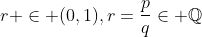,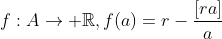(where A is natrual number larger than 1) maximized at the second crossings. Why?

Definition. Let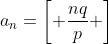be the largest integer that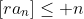.

The above claim is equivalent to claiming that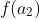is the global maximum of f.

Theorem.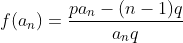.

Proof.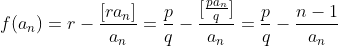, the result follows.

Theorem.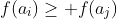iff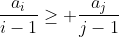.

Proof. Straightforward by direct expansion.

Theorem.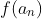maximized at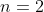.

Proof. This is equivalent to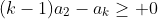.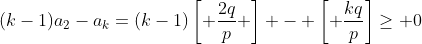which can be shown using modular arithmetic. (It isn't hard to check: there are only finite case to check.)

How about the case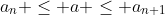?

Note that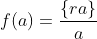and let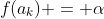. Consider the case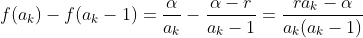which is larger than zero for large enough a_k. Now we can summarize our approach to find our optimal solution:

Check the following value of a: if they are a better solution replace the current one.
1) The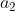(usually the best answer)
2) Find smallest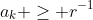. For each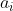find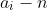so that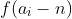is maximized in the range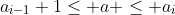.

Complexity? Something like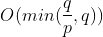. The problem is how often the answer falls onand how should we make the algorithm more efficient? I would leave that part open.

## Sunday, 19 May 2013

### A number theory problem from online games Part 1

The situation comes from online game in a very natrual style.

Unlike modern banking system, the statistics (like money) in online games are usually integer, but that makes some problem (to the producer sometimes, and sometimes to players) in particular the statistic conversion.

Like, we are obtaining energy A from energy B at a rate of 36.5%. It is natrual that it takes 365 units of B for us to get 1000 units of A. For what if we want 1 units of A? 3 units?

In the game that I am playing they take the rounding off approach --- of course --- this is beneficial if we goes B->A but not-so-good when we go in another way. For example 1 unit of A is supposed to take 0.365 units of B and now it's rounded off to zero. 3 units of A takes 1 unit of B to convert with. Of course, the system won't let you to obtain 1 unit of A 'with zero unit of B' --- you need at least one unit of input.

The problem comes: in what ratio (in particular, the amount of A I want every time) so that we maximize our gain (i.e. ratio above the supposed rate)? We can now state our problem:

Instance: A real number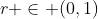Prroblem: Find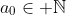to maximize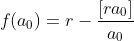.

Well, r causes a bit of trouble when it isn't rational, so we modify the instance a bit:

Instance: A sequence of real number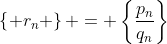that converges to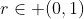. It follows that the sequence is an approximation to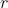that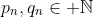and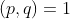. Moreover, it follows that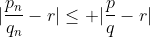for all fractions that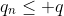. If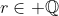, the sequence constantly equal to the fraction that equals to.

We should also specify that we can't convert to something (larger than zero) using zero resources. So the problem becomes:

Problem: Find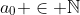to maximize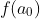as defined above, with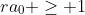.

Where should we start with? It is clear that when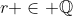the problem can be easily solved. To see this we shall consider rational instance for the rest of (this part of) the passage with the notation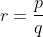.

Lemma. For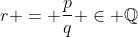,.

Proof. For, there is a multiplicative inverse of p mod q. Let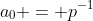in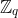, it is clear that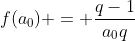.

For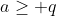,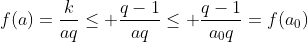.

It's like that the above lemma gives us a possible answer to the problem, but is it the best answer? Let's divide it in two cases:

Theorem. If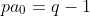, then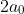is the optimal answer.

Proof. It is clear that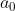can't be a valid answer, and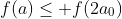for all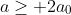using similar arguement with the lemma. To see thatfor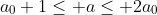, notice that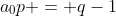so that the integer part of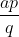do not increase in the given range, the result immediately follows.

Similarly, if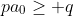we check all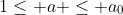to get the optimal answer. It follows that the optimal answer is at mostusing the similar arguement.

If we want to be faster, check all crossing and the corresponding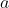value so that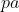is just under the multiple of q, now we should get the answer.

Why 'should'? Think about the situation:

Suppose we getas the 'optimal' answer using the faster way, is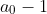a better answer? Is this even possible?... We shall see it shortly...